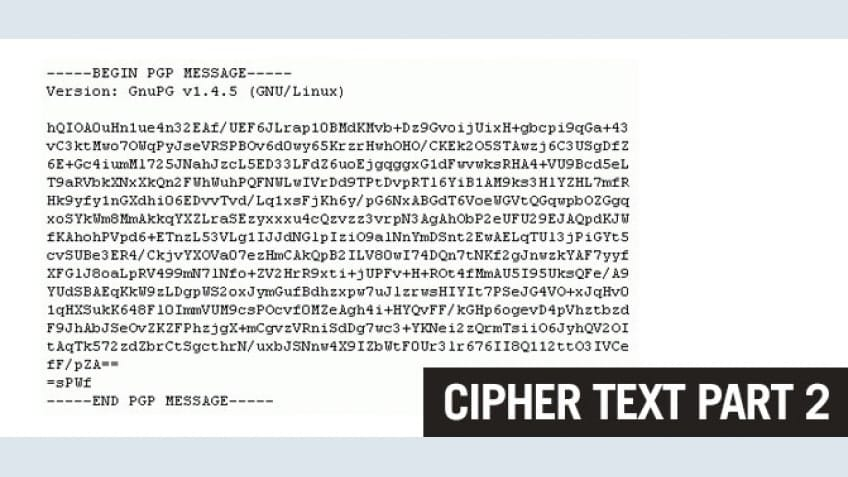## What is Cryptanalysis

Cryptanalysis is the study of methods for obtaining the meaning of encrypted information, without access to the secret information that is typically required to do so. Typically, this involves knowing how the system works and finding a secret key. Cryptanalysis is also referred to as codebreaking or cracking the code. The ciphertext is generally the easiest part of a cryptosystem to obtain and, therefore, is an important part of cryptanalysis. Depending on what information is available and what type of cipher is being analyzed, cryptanalysts can follow one or more attack models to crack a cipher.

## Historical Ciphers

Historical pen and paper ciphers used in the past are sometimes known as classical ciphers. They include:

• Substitution Cipher: The units of plaintext are replaced with ciphertext (Caesar cipher and One-time pad)
• Transposition Cipher: The ciphertext is a permutation of the plaintext (Rail fence cipher)
• Polyalphabetic substitution Cipher: a substitution cipher using multiple substitution alphabets (Vigenère cipher and Enigma machine)
• Permutation Cipher: a transposition cipher in which the key is a permutation

Historical ciphers are not generally used as a standalone encryption solution because they are quite easy to crack. Many of the classical ciphers can be broken using brute force or by analyzing the only ciphertext except the one-time pad.

## What is Substitution Cipher

Substitution of single letters separately—simple substitution—can be demonstrated by writing out the alphabet in some order to represent the substitution. This is termed a substitution alphabet. The cipher alphabet may be shifted or reversed (creating the Caesar and Atbash ciphers, respectively) or scrambled in a more complex fashion, in which case it is called a mixed alphabet or deranged alphabet. Traditionally, mixed alphabets may be created by first writing out a keyword, removing repeated letters in it rather than writing all the remaining letters in the alphabet in the usual order.

Using this system, the keyword "zebras" gives us the following alphabets:

 Plaintext alphabet: ABCDEFGHIJKLMNOPQRSTUVWXYZ Ciphertext alphabet: ZEBRASCDFGHIJKLMNOPQTUVWXY

## What is Transposition Cipher

In cryptography, a transposition cipher is a method of encryption in which the positions held by units of plaintext (which are commonly characters or groups of characters) are shifted according to a regular system so that the ciphertext constitutes a permutation of the plaintext. That is, the order of the units is changed. Mathematically a bijective function is used on the characters' positions to encrypt and an inverse function to decrypt.

## What is Block Cipher

In cryptography, a block cipher is a deterministic algorithm operating on fixed-length groups of bits, called blocks, with an unvarying transformation that is specified by a symmetric key. Block ciphers are important elementary components in the design of many cryptographic protocols and are widely used to implement encryption of bulk data.

## What is Stream Cipher

In cryptography, a stream cipher is a symmetric key cipher in which plaintext digits are combined with a pseudorandom cipher digit stream (keystream). In a stream cipher, each plaintext digit is encrypted one at a time with the corresponding digit of the keystream, to give a digit of the ciphertext stream. An alternative name is a state cipher, as the encryption of each digit is dependent on the current state. In practice, a digit is typically a bit and the combining operation an exclusive-or (xor).

In cryptanalysis and computer security, password cracking is the process of recovering passwords from data that have been stored in or transmitted by a computer system. A common approach is to try guesses for the password repeatedly. Another common approach is to say that you have "forgotten" the password and then change it.

### Reference:

WikipediaSimplilearn